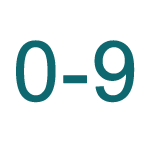Report a Problem
Suggestions

#Base10 To Binary, Octal, Hex Converter

Last updated: Saturday, April 29, 2023

The base10 number system, also known as the decimal system, is the most widely used number system in the world. It consists of 10 digits (0-9), and each digit represents a value that is a power of 10. For example, the number 352 in base10 represents 3 x 10^2 + 5 x 10^1 + 2 x 10^0.

The binary number system, on the other hand, uses only two digits (0 and 1) to represent numbers. Each digit in a binary number represents a power of 2. For example, the binary number 1010 represents 1 x 2^3 + 0 x 2^2 + 1 x 2^1 + 0 x 2^0, which equals 10 in base10.

The octal number system uses eight digits (0-7) to represent numbers, and each digit represents a power of 8. For example, the octal number 27 represents 2 x 8^1 + 7 x 8^0, which equals 23 in base10.

The hexadecimal number system uses 16 digits (0-9 and A-F) to represent numbers, and each digit represents a power of 16. For example, the hexadecimal number 3A represents 3 x 16^1 + 10 x 16^0, which equals 58 in base10.

Please enter a binary number (Base 2 Numeral)
Please enter an octal number (Base 8 Numeral)
Please enter a hex number (Base 16 Numeral)
Bookmark this page or risk going on a digital treasure hunt again